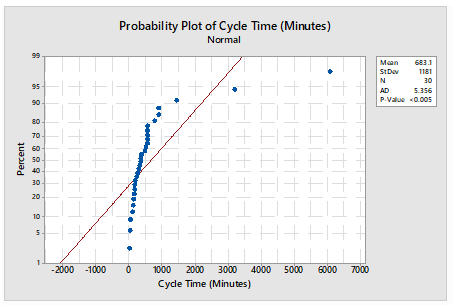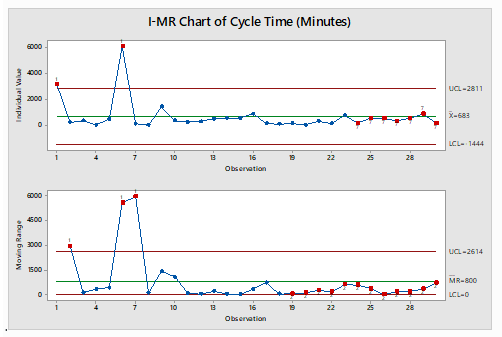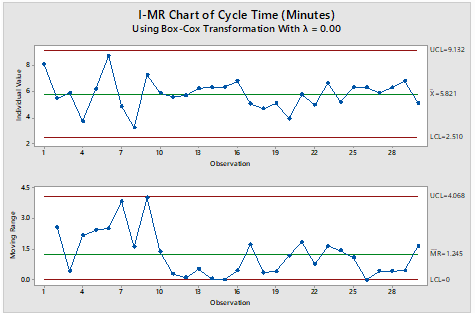• 0

# Control Charts with Transformed Data

Control Chart is a graphical tool used to determine process performance with time. These were developed by Walter A. Shewhart and hence are also known as Shewhart charts. These charts help determine whether the process is stable or not. If the process is stable, the process will only have inherent variation (Common Cause variation). The region of common cause variation is given by Control limits (Upper and Lower control limits). Any process variation outside these limits is considered as Special Cause Variation

Data Transformation is a method to transform non-normal data to normal thus allowing the application of statistical tools on the normalized data

An application-oriented question on the topic along with responses can be seen below. The best answer was provided by Vijay Tomar on 6th Jun 2023.

Applause for all the respondents - Khalandar S, Moushmi Kandori, Gitarchana Roy, Raghavendra Rao Althar, Vidhya Rathinavelu, Vijay Tomar, Partho Karmakar.

## Question

Q 570. IMR Control charts for continuous data work on the assumption that data follows normal distribution. However, in the real world data seldom follows normal distribution. How useful is the check for process stability using transformed data? Provide relevant examples to support your answer.

Note for website visitors -

## Recommended Posts

• 0

An I-MR Chart  is a control chart which is used when data is in continuous category and is collected once at a time. It consists of two charts placed one over above, I Chart which is individual chart and MR chart is plotted for moving range which is absolute value of the difference between two consecutive points.

Data following normal distribution is an assumption while drawing I-MR chart however in practical or real-world problem data doesn’t follow normal distribution all the time hence Process stability follows major role. I-MR charts are very sensitive to Normality of the data. Non-Normal data if considered as normal data can cause unexpected behaviors including false alarm rates and difficulty in identifying the special cause variation.

If data is not normal, it is always advisable to do transformation using Box-Cox or Johnsons transformation to avoid the false alarm and get the right behavior of data for stability and control.

A normal distribution may have the value from minus to plus infinity.  In the real-world example this doesn’t occur physically very often.  For example, Cycle time cannot be in negative numbers.

Following is the Example for drawn I-MR charts when data is considered as normal however data is not normal and respective I-MR charts using data transformation: -

Cycle time in Minutes: -

 Sl.No Cycle Time (In Minutes) S.No Cycle Time (In Minutes) S.No Cycle Time (In Minutes) 1 3196 11 267 21 322 2 241 12 302 22 147 3 372 13 518 23 774 4 42 14 554 24 185 5 481 15 566 25 556 6 6081 16 900 26 555 7 131 17 158 27 361 8 26 18 109 28 556 9 1445 19 167 29 898 10 363 20 51 30 170

Table 1

Probability Plot of dats Using Mini-tab Normality test for data in Table 1: -

Normality test is done to illustrate whether data is normal or non-normal.I-MR Charts drawn in Minitab for Table 1 mentioned assuming data following Normal distribution: -

The chart clearly illustrates that process is out of control, however out of control points are trigged due to false alarmsI-MR Chart drawn in Minitab for Table 1 mentioned after transforming days using Box-Cox transformation: -
The Chart clearly illustrates that process is in control, our of control data points mentioned earlier were due to false alarm in wrong assumption of data being normal.##### Share on other sites

• 0

The IMR (Individuals and Moving Range) control chart is widely used to check the stability of a process over time and it is true that the IMR chart assumes that the data follows a normal distribution, but in reality, data often deviates from normal distribution.

One approach to resolve such scenario is that data transformation techniques from non-normal to normal using various transformation techniques like Box-Cox transformation, logarithmic and square root transformations. After transforming the data, we can plot a control chart and check for process stability. But the limitation of this approach is that some transformations may not work well for certain types of data (If data set is either not well represented or contain outliers) which led to bias.

Therefore, it is recommended to use transformed control charts as a replacement for traditional control charts. Transformed control charts can be useful in detecting process instability when normality assumptions are violated. However, it's important to note that transforming the data doesn't always guarantee that it will follow a normal distribution. Additionally, if the underlying process is non-normal, using a transformed control chart may not accurately reflect the true process behavior. In conclusion, using transformed data can be a useful tool for checking process stability when the underlying data is non-normal

Some practical examples of transformed control charts are:

1. Manufacturing: A chemical manufacturing plant wants to monitor the concentration of a certain chemical in their production process. However, the data is highly skewed and does not follow a normal distribution. To address this, they apply a logarithmic transformation to the data and use an X-bar chart with transformed data to monitor the process mean over time.

2. Service: A software development team wants to monitor the number of bugs found in their code during each sprint. However, the data is highly skewed and does not follow a normal distribution. To address this, they apply a Box-Cox transformation to the data and use an individuals chart with transformed data to monitor the process variability over time.

In each of these examples, transforming the data allows for more accurate monitoring of the process over time using control charts. It's important to note that choosing an appropriate transformation requires careful consideration of the underlying statistical properties of the data, as well as any practical implications for interpreting and acting on the results.

##### Share on other sites

• 0

Using IMR control charts for continuous data assumes that the data follows a normal distribution. If the data does not follow a normal distribution, using transformed data may be useful in verifying process stability.

Transforming data can help to make the data conform to a normal distribution. For example, if the data is positively skewed, using a logarithmic transformation can help to normalize the data. Similarly, if the data is negatively skewed, a reciprocal or square root transformation may be used.

Using transformed data can be useful in detecting process stability by identifying any patterns or changes in the data that may indicate a shift in the process mean or variability. For instance, if the control chart shows a trend or pattern with transformed data, it may indicate a shift in the process. In this case, further investigation may be required to identify the problem and take corrective action.

For example, consider a manufacturing process that produces steel rods. The process involves heating and cooling the rods to achieve the desired tensile strength. The control chart for the tensile strength of the rods shows a trend with the raw data, indicating a possible shift in the process mean. However, after applying a logarithmic transformation to the data, the control chart shows no trend or pattern. This suggests that the process is stable and the raw data is not normally distributed.

In another example, consider a call center that tracks the number of calls received per hour. The control chart for the call volume shows a shift in the process mean with the raw data, indicating a possible problem. However, after applying a square root transformation to the data, the control chart shows no shift in the process mean. This suggests that the process is stable and any concerns were due to the non-normal distribution of the raw data.

In conclusion, while IMR control charts for continuous data are designed for normal distribution, transformed data can be useful in identifying process stability. If the transformed data shows no patterns or trends, it suggests that the process is stable, regardless of the distribution of the raw data. However, it is important to note that transformations should be used with caution, and the results should be interpreted carefully to avoid incorrect conclusions.

##### Share on other sites

• 0

IMR Control Charts is the most commonly used control charts which is a combination of the Individual and Moving range chart which helps us to analyse process stability and variability. It is used when we analyse continuous data and the data is normal.

It is true that in the real world we seldom find normal data. In case of IMR charts, results will still be valid in case the data is moderately normal.

The data can be transformed in case the data is non normal and highly skewed. The transformations can be done using either  Box Cox Transformation, Logarithmic or Johnson’s Transformation.

The data can be transformed and then appropriate control charts can be used to check on process stability

Example:-

1.    Usually time , effort and defect related data is non normal because the data can spread from 0 to infinite.

One should investigate the data collection process and if MSA was done correctly before trying to transform data. Data transformation should be the last resort.

Non parametric tests can also be used with non normal data. They usually analyse the median instead of the mean. However, it is possible that non parametric tests might not show the minor differences. In such cases data transformation is the only option left.

##### Share on other sites

• 0

If the data is not normal and is transformed, then that impacts the accuracy and validity of the control charts if in case they are to be monitored. This makes it important to handle the transformation of data in a more cautious way. There are no particular method of transformation that fits a particular scenario. Different method of transformation have different benefits. Some of the key considerations while choosing transformational techniques are, extent of non-normality prevalent in the data, how easy it is to apply the method, how much better is the interpretability of the data, how well the transformation supports the process performance and other factors. Its worthwhile to give due consideration of non-parametric method before considering the data transformation. Median charts, run charts, Weibull distributions are few methods that fit into non-parametric methods. Data smoothing is an approach that can assist in removal of noise from the data, clustering, binning and regression methods are part of noise removal approach. Data generalization is another approach of data transformation, where low level attributes are transformed to high level attributes. This is suitable in case if there are finite number of values in data that have large number of distinct values. Like in case of data set that has data for city, street, country and state, we can define hierarchy of these attributes by ordering them with respect to their relations. Order would be country, state, city and street. These are couple of customized data transformation as needed for specific scenario.

##### Share on other sites

• 0

In real world, the data rarely follows a normal distribution. Data is affected by outliers and measurement errors, because of which IMR chart may not be effective in detecting changes, as these charts assume that the data is normal.

In such cases, we can use charts for non normal data or we can transform the data, depending on the scenario and the data type. Data transformation techniques, such as the Box-Cox or Johnson transformation, can help stabilize variances and make the data more symmetric, allowing for more accurate interpretations and applications of control charts.

Data can also be split into rational sub groups and then buliding control charts on each split data.

Checking for process stability using transformed data helps to identify potential problems in a process. By transforming the data, we can highlight any trends or patterns that might not be visible in the raw data. This can help us to identify potential causes of variation. Then corrective actions can be identified to improve the stability of the process and prevent any problems from re-occurring.

Example: A call center will monitor the waiting times in the queue. If the data is not normal due to variability, then the distribution will be a non normal distribution and IMR chart may not accurately detect the actual problem or the trends. If the wait time data can be transformed, then the analysis on the data will be more accurate and will help the call center to take effective decisions.

##### Share on other sites

• 0

The assumption of normality in IMR (Individuals and Moving Range) control charts is indeed a common practice. However, it is true that in the real world, data often deviates from a perfect normal distribution. While transformed data can be used to address non-normality to some extent, its usefulness in checking process stability depends on various factors, including the nature of the transformation applied and the specific characteristics of the data.

Transforming data involves applying mathematical operations to the original dataset to achieve a more desirable distribution or meet certain assumptions. Some common transformations include logarithmic, square root, and Box-Cox transformations. These transformations can help make the data more symmetrical and reduce skewness or variability.

To illustrate the usefulness of transformed data for checking process stability, let's consider an example with 20 data points. Suppose we have collected data on the time it takes for a process to complete a certain task in min. Here are the analysis original data points:

AHT1     25          30          32          31          29          27          30          28          33              26          24          28          34          40          45          42          50          38              35          30

First, lets perform the normality test and create an IMR control chart using the original data. Control chart was plotted using these data points and analyze the process stability based on the control limits and the presence of any out-of-control points

Next, let's apply Logarithmic transformation to the data, perform the normality test and create a new control chart. The transformed data points are as follows:

LOG AHT1          1.40       1.48       1.51       1.49       1.46       1.43       1.48              1.45       1.52       1.41       1.38       1.45       1.53       1.60       1.65              1.62       1.70       1.58       1.54       1.48

Interpretaion:

By analyzing both control charts, we can assess the process stability based on the control limits and patterns in the data points. If the data points fall within the control limits, show no significant patterns, and exhibit randomness, the process is considered stable.

In this example, both the raw and transformed data control charts show data points with same pattern. Therefore, we can conclude that there is no change in the process stability, even though the raw data was more skewed than the transformed data. The transformation allowed us to assess the process stability accurately. In this examples , though the moving range suggests process stability, one needs to understand the reason for shift in pattern along with the one data point that failed at point 17 in the individual chart.

The point to remember is that  the appropriateness of the transformation and the resulting interpretation depends on the specific context and understanding of the underlying process. It's always recommended to consult with subject matter experts and consider additional analyses if needed.

In order to utilize an I-MR Chart, there is no mandatory requirement for  the data to be always normal distributed, however   extremely skewed data can cause some unwanted outcome including high false-alarm rates. If data appears skewed, we can investigate to see whether that is an indication of an out of control process or as per expectations for this  type of process, and if expected, we can look for transformation of the data.

##### Share on other sites

• 0

Vijay Tomar has provided the best answer to this question by elaborating the IMR chart and also providing an example of working with Control Chart for transformed data.

## Create an account

Register a new account

• ### Who's Online (See full list)

• There are no registered users currently online
• ### Forum Statistics

• Total Topics
3.2k
• Total Posts
16.4k
• ### Member Statistics

• Total Members
54,882
• Most Online
990